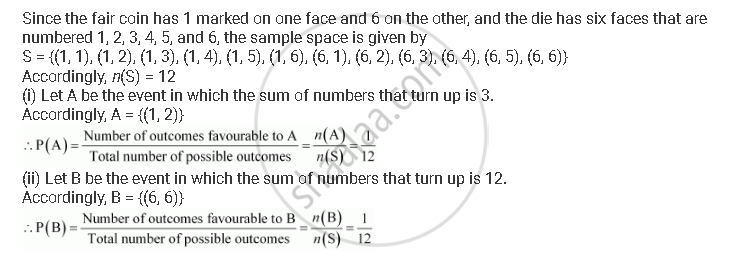CBSE (Arts) Class 11CBSE
Share

# A Fair Coin with 1 Marked on One Face and 6 on the Other and a Fair Die Are Both Tossed. Find the Probability that the Sum of Numbers that Turn up is (I) 3 (Ii) 12 - CBSE (Arts) Class 11 - Mathematics

ConceptAxiomatic Approach to Probability

#### Question

A fair coin with 1 marked on one face and 6 on the other and a fair die are both tossed. Find the probability that the sum of numbers that turn up is (i) 3 (ii) 12

#### SolutionIs there an error in this question or solution?

#### Video TutorialsVIEW ALL 

Solution A Fair Coin with 1 Marked on One Face and 6 on the Other and a Fair Die Are Both Tossed. Find the Probability that the Sum of Numbers that Turn up is (I) 3 (Ii) 12 Concept: Axiomatic Approach to Probability.
S